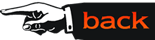## Algebraic Structures and Number Theory

The Fundamental Theorem of Arithmetic (FTA) and related notions such as greatest common divisor (GCD) and least common multiple (LCM) are fundamental in algebra and number theory. It is important that middle grade mathematics teachers reach a firm understanding of these concepts through various problem solving experiences in mathematical contexts, as well as real world settings.

A typical class period (of our Algebraic Structures course) concerning these topics might begin by dividing the students into small collaborative groups (3 or 4 students per group) and then examining some specific standards-based middle grade (or secondary grade) mathematics curricular materials dealing with these concepts. For example, the sixth grade unit Prime Time from the Connected Math Project, Dale Seymour Publications,1998, introduces and develops many interesting mathematical explorations involving prime numbers, factorization, GCD, LCM, and in general provides a sound framework to help facilitate the understanding and appreciation of the power and utility of FTA.

A particular lesson from Prime Time (Investigation 4.2, Looking at Locust Cycles, page 38) focuses on cicadas (commonly called locusts) having different life cycles and considers several different natural questions related to these cycles. For instance, Problem 4.2 raises the following questions (involving LCM): a) How many years pass between the years when both 13-year and 17-year locusts are out at the same time? Explain how you got your answer. b) Suppose there were 12-year, 14-year, and 16 year locusts, and they all came out this year. How many years will it be before they all come out together? Explain how you got your answer. The discussion on cicadas proceeds with a follow-up problem and is continued, with added complexity, on page 42. For example, after a brief introductory paragraph sets the stage, Extension 7.2 (a), page 42, asks the following question: Suppose cicadas have predators with 2-year cycles. How often would 12-year locusts face their predators? Would life be better for 13-year locusts?

In the process of investigating these materials, the college student will not only directly experience the concepts they themselves will be teaching, but will also have multiple opportunities to connect and deepen their understanding of these notions. In particular, after the individual collaborative groups report on their findings and methods to the whole class, it is then convenient to consider generalities and computational tools for analyzing similar problems in more varied situations. For instance, the following collection of ideas (along with other related notions) naturally links to the mathematics encountered in this specific middle school unit:

• expressing the GCD of two integers and LCM of two integers in terms of the prime factors of the given integers;
• determining the relationship between the GCD and LCM of two integers;
• using and proving Euclids algorithm to compute the GCD and the LCM using the discovered relationship) of two arbitrary integers;
• employing the computations of Euclids algorithm to write the GCD of two integers as a linear combination of the two integers;
• deriving several elementary number theoretic facts by exploiting the linear combination identity.

The progression of these ideas would occur over several class periods and would also be integrated into other points of study throughout the course.

The power and versatility of the symbolic algebra/graphing calculator will be fully exploited throughout the course, not only in computational venues, but especially in mathematical situations involving exploration, conjecture, discovery, and the construction of numerical or algebraic proofs. Questions arising on how the calculator performs its tasks will lead to important discussions on the nature of algorithms (such as Euclids algorithm) and help provide a fundamental understanding of their role in mathematics.